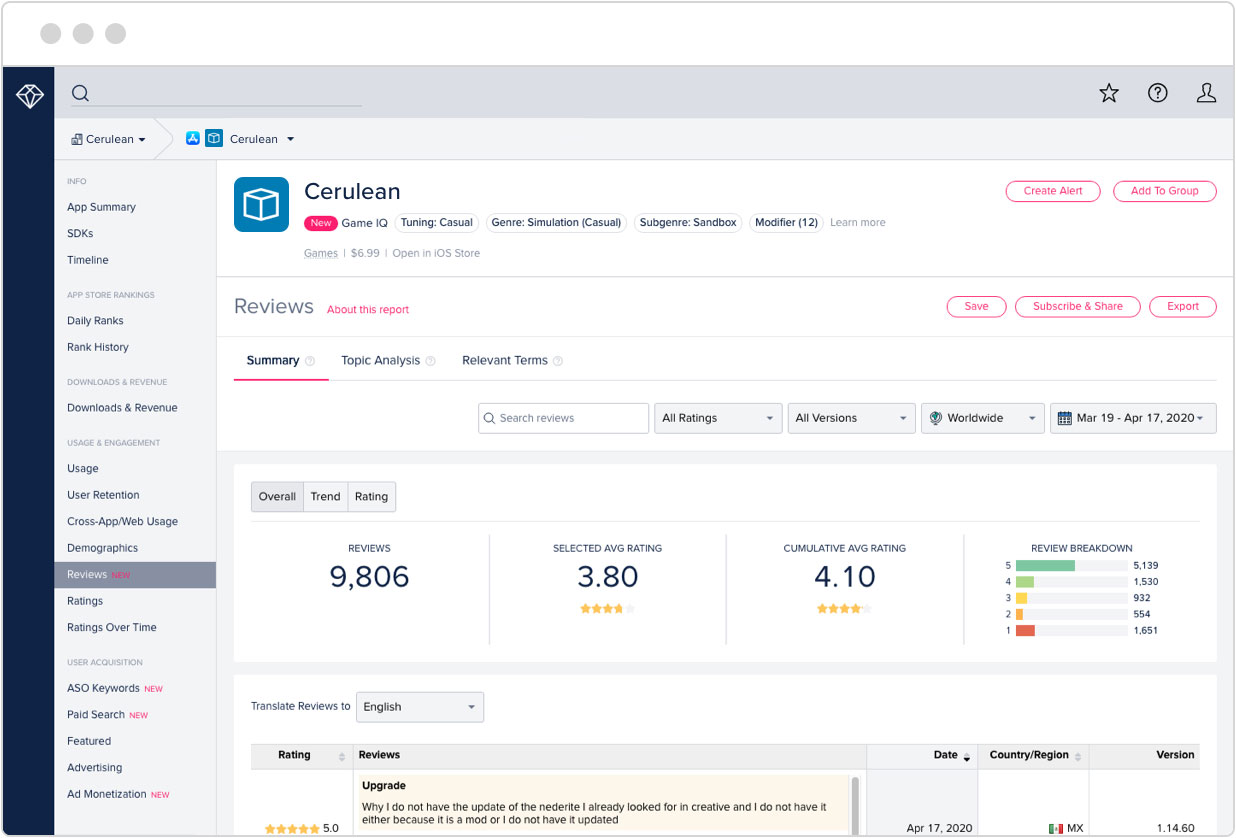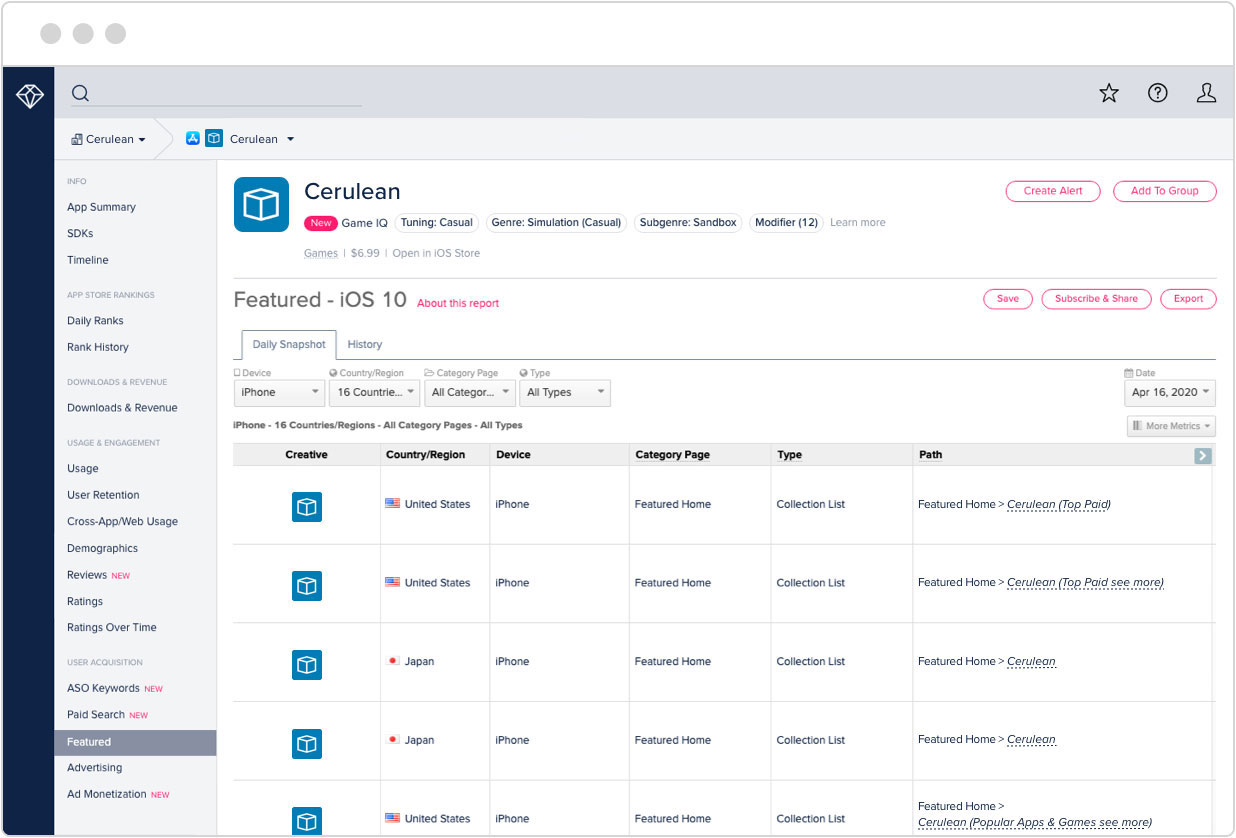# iformula pro X

### 免费注册来发掘更多！### 应用评价和评级### 应用说明

iformula pro X is an intuitive higher mathematic formula equations library, with all math topics you need to do your math work. It is full searchable, well illustrated and helps you to learn new math equations or to remember equations fast and effective you have learned years before.

*** We are the world leading developer for higher math formula, equation apps on iPhone and iPad (iformula pro, iformula pro HD), now we are proud to present our equivalent version for the mac ! ***

It assists you with your higher math homework for your math high school course or for university, or to solve math problems or to support you writing your thesis or doctoral dissertation or just for exercising !

Searching the Internet for hours to find a simply equation belongs to the past, iformula pro X includes all math topics you need !

► Math topics:
* Algebra
* Analytic Geometry
* Math Basics
* Complex numbers
* Differential, Integral, Calculus
* Statistics, Probability
* Series, Sequences
* and much more

► Scientific calculator in separate window
(which, for example allows you to store values and remember them even after the restart of the software or your Mac !)

► Level indication for your math skill is included (stars)

► Note system to store important information for each equation page (notes are saved permanently)

► Print out what you see (print your math topic), for further processing (e.g. at the classroom, office, lecture hall, exams)